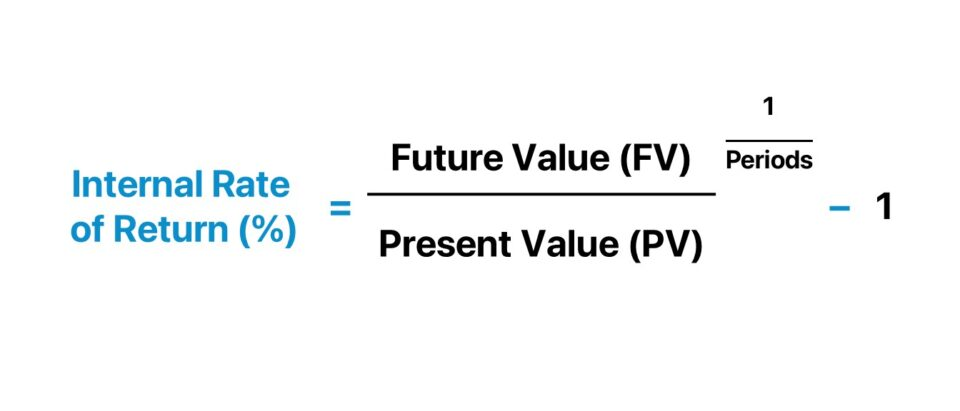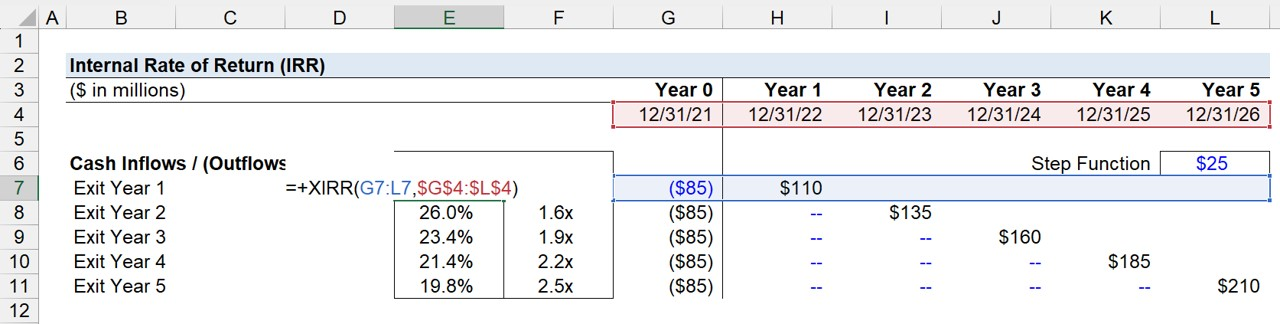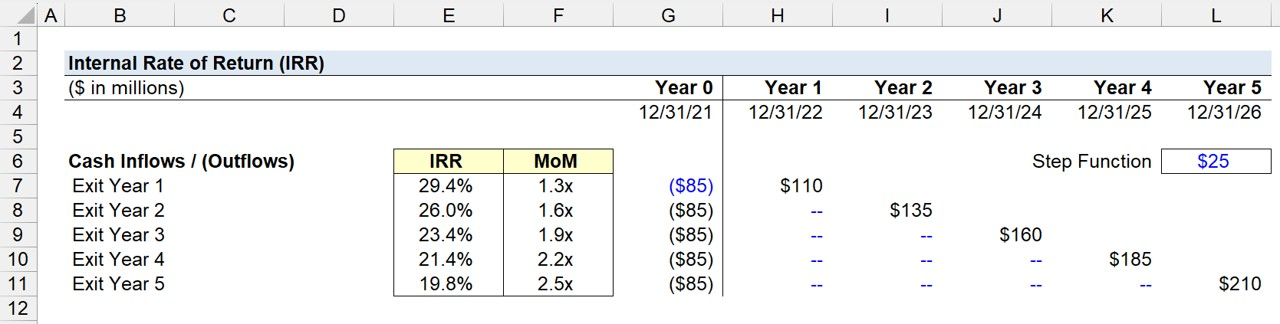Welcome to Wall Street Prep! Use code at checkout for 15% off.# Internal Rate of Return (IRR)

Guide to Understanding Internal Rate of Return (IRR)## How to Calculate Internal Rate of Return (IRR)?

The internal rate of return (IRR) metric estimates the annualized rate of return that an investment is going to yield.

Unlike the multiple of money (MoM), another metric tracked by investors to measure their returns, the IRR is considered to be “time-weighted” because it accounts for the specific dates that the cash proceeds are received.

The higher the internal rate of return (IRR), the more profitable a potential investment will likely be if undertaken, all else being equal.

The manual calculation of the IRR metric involves the following steps:

1. The future value (FV) is divided by the present value (PV)
2. The amount is raised to the inverse power of the number of periods (i.e., 1 ÷ n)
3. From the resulting figure, one is subtracted to compute the IRR

## Internal Rate of Return Formula (IRR)

The formula for calculating the internal rate of return (IRR) is as follows:

Internal Rate of Return (IRR) = (Future Value ÷ Present Value)^(1 ÷ Number of Periods) 1

Conceptually, the IRR can also be thought of as the rate of return wherein the NPV of the project or investment equals zero.

The alternative formulas, most often taught in academia, involve backing out the IRR for the equation to hold true (and require using a financial calculator).

• 0 = CF t = 0 + [CF t = 1 ÷ (1 + IRR)] + [CF t = 2 ÷ (1 + IRR)^2] + … + [CF t = n ÷ (1 + IRR)^ n]

Or, an alternative method to calculate the IRR is the following:

• 0 = NPV Σ CF n ÷ (1 + IRR)^ n

## XIRR vs. IRR Excel Function: What is the Difference?

The difference between using the XIRR and IRR function in Excel is as follows.

• XIRR Function in Excel → The Excel XIRR function is preferable over the IRR function as it has more flexibility by not being restricted to annual periods. Under XIRR, daily compounding is assumed, and the effective annual rate is returned. But for the IRR function, the interest rate is returned assuming a stream of equally spaced cash flows.
• IRR Function in Excel → The drawback to the Excel IRR function is the implicit assumption that each cell is separated by precisely twelve months. Unlike the IRR Function in Excel, the XIRR function can handle complex scenarios that necessitate taking into account the timing of each cash inflow and outflow (i.e. the volatility of multiple cash flows).

## What are the Limitations to Internal Rate of Return (IRR)?

The internal rate of return (IRR) cannot be singularly used to make an investment decision, as in the case of most financial metrics.

• Cash Flow Timing: The internal rate of return (IRR) metric is imperfect and cannot be used as a standalone measure due to being highly sensitive to the timing of the cash flows. Thus, the IRR can potentially be misleading in its portrayal of returns under certain circumstances wherein a greater proportion of the cash flows are received earlier.
• Shorter Holding Periods: The implied IRR from an investment could be impressively high, yet stem from the shorter holding period, which caused the returns to be artificially inflated and unsustainable if the holding period were to be hypothetically extended longer.
• Dividend Recap: If a private equity firm were to issue itself a dividend soon after a leveraged buyout (LBO), i.e. a dividend recapitalization (or recap), the payment would increase the IRR to the fund regardless of whether the multiple-of-money (MoM) meets the required returns hurdles – which can cause the IRR to be potentially misleading here.

## What is a Good IRR?

In the context of a leveraged buyout (LBO) transaction, the minimum IRR is usually 20%. However, the IRR can be easily distorted by the earlier receipt of cash proceeds.

For instance, suppose a private equity firm (PE) anticipates an LBO to yield an 30% internal rate of return (IRR) if sold on the present date, which at first glance sounds great.

But from a more in-depth look, if the multiple on money (MoM) – i.e. the multiple on invested capital (MOIC) – on the same investment is merely 1.5x MoM, that would cause the implied return to be far less impressive.

The 30% IRR is attributed more towards a quicker return of capital, rather than substantial growth in the size of the investment.

Nonetheless, the IRR tends to be the most benchmarked marketing metric for the performance of investment funds and influential with regard to the ability for firms to meet (or surpass) their capital raising efforts for their next fund from existing and new limited partners (LPs). Because of that factor, most firms pay a significant amount of attention to their fund’s IRR.

## What Causes IRR to Increase or Decrease?

The following factors are the main contributors which drive the internal rate of return (IRR):

 Positive IRR Levers Negative IRR Levers Earlier Extraction of Exit Proceeds (e.g., Dividend Recapitalization, Monitoring Fees) Delayed Receipt of Exit Proceeds (e.g., Sale Delay Caused by Lack of Interested Buyers) Increased Free Cash Flows from Strong Revenue and EBITDA Growth Reduced Free Cash Flows (FCFs) and Profit Margins Multiple Expansion (i.e. Exiting at a Higher Multiple than the Entry Multiple) Multiple Contraction (i.e., Lower Exit Multiple than Purchase Multiple)

Regardless, the internal rate of return (IRR) and MoM are both different pieces of the same puzzle, and each comes with its respective shortcomings.

Of course, the magnitude by which an investment grows matters, however, the pace at which the growth was achieved is just as important.

## IRR Calculator

We’ll now move to a modeling exercise, which you can access by filling out the form below.#### Excel TemplateSubmitting...

## 1. LBO Model Entry Assumptions

Suppose a private equity firm made an equity investment of \$85 million in 2022 (Year 0).

• Year 0 = –\$85 million Cash Outflow

The value of the initial investment stays unchanged regardless of which year the firm exits the investment.

Since the investment represents an outflow of cash, we’ll place a negative sign in front of the figure in Excel.

## 2. Cash Flow Analysis Example

Afterward, the positive cash inflows related to the exit represent the proceeds distributed to the investor following the sale of the investment (i.e. realization at exit).

Here, our simple assumption is that the exit proceeds will increase by a fixed amount of \$25 million each year, starting from the initial investment amount of \$85 million.

• Annual Growth in Sponsor Proceeds (\$) = +\$25 million per Year

Therefore, the exit proceeds in Year 1 are \$110 million while in Year 3, the proceeds come out to \$160 million.

## 3. IRR Calculation Example

Once our table depicting the cash outflow in Year 0 (the initial investment) and the cash inflows (the exit proceeds) at different dates in the holding period is done, we can calculate the IRR and MoM metrics from this particular investment.

To determine the internal rate of return (IRR) on the LBO investment in Excel, follow the steps below.

1. Start by listing out the value of all the cash inflows/(outflows) and the corresponding dates of the date of receipt
2. Use the XIRR Excel function (“= XIRR (Range of Cash Flows, Range of Timing)”); the first input requires you to drag the selection box across the range of cash inflows/(outflows)
3. For the second input, do the same across all the corresponding dates.
4. Press Enter to Calculate the Internal Rate of Return (IRR)

For example, if the exit year is assumed to be Year 1, the IRR comes out to 29.4%.

=XIRR(G7:L7,\$G\$4:\$L\$4)To reiterate from earlier, the initial cash outflow (i.e. sponsor’s equity contribution at purchase) must be entered as a negative number since the investment is an “outflow” of cash.

Note that for the formula to work and be dragged down, the date selection must be anchored in Excel, i.e. fixed.

While the two main factors are the entry investment and exit sale proceeds, other inflows such as dividends or monitoring fees (i.e. the services related to portfolio company consulting) must be input as positives, as well as any additional equity injections later on in the holding period.

## 4. Multiple of Money Calculation Example (MoM)

In order to calculate the multiple-of-money (MoM), we’ll calculate the sum of all the positive cash inflows from each holding period.

We must then divide that amount by the cash outflow in Year 0.

For instance, assuming a Year 5 exit, the exit proceeds of \$210 million are divided by -\$85 million to get an MoM of 2.5x.

• Multiple-of-Money (MoM) = \$210 million ÷ (\$85 million) = 2.5x

## 5. LBO Returns Analysis Schedule (IRR and MoM)

In the final section of our IRR calculation tutorial in Excel, we’ll compute the IRR for each exit year period using the XIRR Excel function.

Based on the completed output for our exercise, we can see the implied IRR and MoM at a Year 5 exit – the standard holding period assumption in most LBO models – is 19.8% and 2.5x, respectively.

• IRR – Exit Year 1 = 29.4%
• IRR – Exit Year 2 = 26.0%
• IRR – Exit Year 3 = 23.4%
• IRR – Exit Year 4 = 21.4%
• IRR – Exit Year 5 = 19.8%

If we were to calculate the IRR using a calculator, the formula would take the future value (\$210 million) and divide by the present value (-\$85 million) and raise it to the inverse number of periods (1 ÷ 5 Years), and then subtract out one – which again gets us 19.8% for the Year 5 internal rate of return (IRR).Master LBO Modeling Our Advanced LBO Modeling course will teach you how to build a comprehensive LBO model and give you the confidence to ace the finance interview.Inline FeedbacksPleasant
August 14, 2023 3:35 am

I have a problem with this irr
I get decimals instead of percentageAsutosh Sabat
February 8, 2023 12:32 am

The cash flow her means operating cash flows only or total cash flows ??February 8, 2023 10:30 am

Hi, Asutosh, In this case, the cash flows are the initial equity investment and the final payout to equity holders at the end, so only cash flows at the beginning and the end. We assume the operating cash flows along the way are used to pay down debt, so what… Read more »Austin Vandersteen
September 7, 2022 9:29 pm

How does the \$25M assumption happen? Where is that assumption from/based in?February 8, 2023 10:31 am

Hi, Austin,

The \$25mm assumption here is to illustrate the idea that the value of the business grows each year (probably due to growth in EBITDA), so if you sell it in a later year, you will sell for a higher price.

BBGreg Kleehammer
January 18, 2022 6:34 pm

Re: IRR   For the calculation of IRR denoted on the IRR & MoM Calculation worksheet and explained in the Internal Rate of Return (IRR) document, are the Cash Flows in Years1 through Year 5 Pre-tax or After-tax? Example: Let’s assume that the \$85m is the full cash investment. If the… Read more »Jeff Schmidt
January 19, 2022 12:29 pm

Greg:

You don’t tax affect the cash investment… that’s literally the amount invested. The cash inflows should be after-tax.

Best,
Jeff

X

The Wall Street Prep Quicklesson Series

7 Free Financial Modeling Lessons

Get instant access to video lessons taught by experienced investment bankers. Learn financial statement modeling, DCF, M&A, LBO, Comps and Excel shortcuts.